# VBA Boolean Data Type (Dim Variable)

## Boolean Variable Type

The VBA Boolean data type is used to store True or False values.   True can also be represented by 1 and False by 0.

To declare an Boolean variable, you use the Dim Statement (short for Dimension):

``````Dim blnA as Boolean
``````

Then, to assign a value to a variable, you simply use the equal sign:

``blnA = True``

When you put this in a procedure, it could look like this:

``````Sub blnExample()
'declare the boolean variable
Dim blnA as Boolean
'run code to populate the variable - usually the code is an if or a case statement
If Range("A1") > 0 then
blnA = true
Else
blnA = False
End If
'show the message box
MsgBox "The test to see if the cell has a value greater than 0 is " & blnA
End Sub``````

If you run the code above, the following message box will be shown.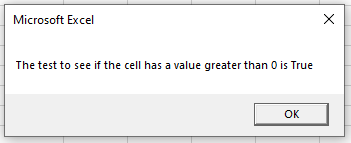## Declare Boolean Variable at Module or Global Level

In the previous example, we’ve declared the Boolean variable within a procedure. Variables declared with a procedure can only be used within that procedure.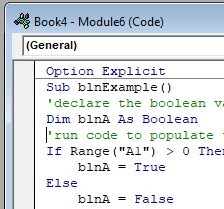Instead, you can declare Boolean variables at the module or global level.

### Module Level

You declare Module level variables at the top of code modules with the Dim statement.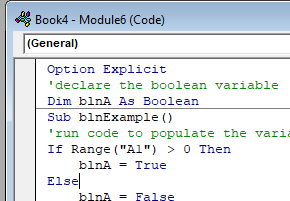These variables can be used with any procedure in that code module.

### Global Level

You also declare Global level variables at the top of code modules. However, instead of using the Dim statement, you would use the Public statement to indicate that the Boolean variable is available to be used throughout your VBA Project.

``Public blnA as Boolean``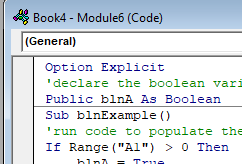If you were to declare the Boolean variable at a module level and then try to use it in a different module, you would get an error.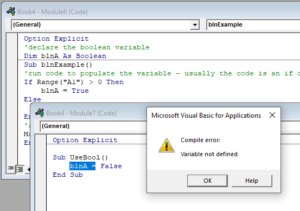However, if you had used the Public keyword to declare the Boolean variable, the error would not occur and the procedure would run perfectly.

## Using a Boolean Variable

You use the Boolean variable in logical comparison.  These are often used with If statements to test if a condition is True or False as per the example above, or in a line of code to apply a logical test – perhaps to see if one value is greater than another.

``````Sub blnExample()
'declare the boolean variable
Dim blnA As Boolean
'test to see if one number is greater than the next number
blnA = 45 > 68
'show the message box
MsgBox blnA
End Sub``````

If you run the code above, you will get the following message box.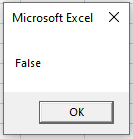because of course 45 is not greater than 68!

## Using Boolean Operators

As Boolean variables are used in logical comparison, we can use the logical operators AND and OR to test to see if more than one condition is true or false.

Stop searching for VBA code online. Learn more about AutoMacro - A VBA Code Builder that allows beginners to code procedures from scratch with minimal coding knowledge and with many time-saving features for all users!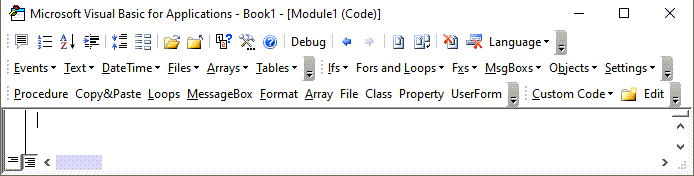### Using the AND operator

We can use the AND function to see if BOTH conditions are met.

``````Sub blnExample()
'declare the boolean variable
Dim blnA As Boolean
'use the AND operator to test to see if both conditions are true
blnA = 10 > 13 And 15 > 12
'show the message box
MsgBox blnA
End Sub``````

or we could run the same test using an If Statement:

``````Sub blnExample()
'declare the boolean variable
Dim blnA As Boolean
'use the AND operator to test to see if both conditions are true
If 10 > 13 And 15 > 12 Then
blnA = True
Else
blnA = False
End If
'show the message box
MsgBox blnA
End Sub``````

Both examples above would return FALSE due to the fact that 10 is NOT greater than 13 – and BOTH conditions have to be True for the Boolean to be True.

### Using the OR operator

We can use the OR function to see if ONE OF the conditions is met.

``````Sub blnExample()
'declare the boolean variable
Dim blnA As Boolean
'use the OR operator to test to see if one of conditions is true
blnA = 10 > 13 Or 15 > 12
'show the message box
MsgBox blnA
End Sub``````

or we could run the same test using an If Statement:

``````Sub blnExample()
'declare the boolean variable
Dim blnA As Boolean
'use the OR operator to test to see if one of conditions is true
If 10 > 13 OR 15 > 12 Then
blnA = True
Else
blnA = False
End If
'show the message box
MsgBox blnA
End Sub``````

These examples would return TRUE due to the fact that 10 is NOT greater than 13 BUT 15 IS greater than 12  – and ONLY ONE condition has to be True for the Boolean to be True.

Using If statements allows us to use more logical operators

### Using the NOT operator

We can also use the NOT operator with the Boolean variable.  The NOT operator negates the value of the condition – so if a condition is true, the NOT operator will return false.

``````Sub FindDifferences()
'declare range variables
Dim rng1 As Range
Dim rng2 As Range
'activate sheet one
Worksheets("Sheet1").Activate
'populate the ranges
Set rng1 = Range("A3")
Set rng2 = Range("B3")
'use the NOT operator to see if the values are equal or not
If Not rng1.Value = rng2.Value Then
MsgBox "The values in the cells are not equal"
Else
MsgBox "The values in the cells are equal"
End If
End Sub``````

VBA Programming | Code Generator does work for you!

## Using the Xor Logical Operator

The Xor logical operator is used to compare two or more conditions.  If one of the conditions is true, it will return TRUE.  If there are 2 conditions, and NEITHER are true or BOTH are true, it will return FALSE.

``````Sub blnExample()
'declare the integers
Dim intA As Integer
Dim intB As Integer
'declare the boolean variable
Dim blnResult As Boolean
'populate the variables
intA = 5
intB = 10
'check to see if one is true
If intA = 5 Xor intB = 5 Then
blnResult = True
Else
blnResult = False
End If
MsgBox blnResult
End Sub``````

In the above example, as ONE of the conditions is TRUE, the message box will return TRUE.

``````Sub blnExample()
'declare the integers
Dim intA As Integer
Dim intB As Integer
'declare the boolean variable
Dim blnResult As Boolean
'populate the variables
intA = 5
intB = 5
'check to see if one is true
If intA = 5 Xor intB = 5 Then
blnResult = True
Else
blnResult = False
End If
MsgBox blnResult
End Sub``````

However, in the example above, as BOTH conditions are true, the message box will return FALSE.

``````Sub blnExample()
'declare the integers
Dim intA As Integer
Dim intB As Integer
'declare the boolean variable
Dim blnResult As Boolean
'populate the variables
intA = 6
intB = 8
'check to see if one is true
If intA = 5 Xor intB = 5 Then
blnResult = True
Else
blnResult = False
End If
MsgBox blnResult
End Sub``````

and finally, as both conditions are FALSE, the message box will also return FALSE.